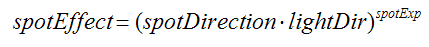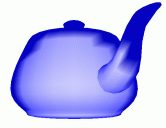Prev: Point Light Per Pixel Next: Simple Texture

This tutorial is based on the previous tutorial as most of the code comes from there. The only thing new in a spot light, when compared to a point light, is that in the former the light rays are restricted to a cone of light where as in the latter the rays are emitted in all directions.

From an OpenGL application point of view the differences between the two are:

• The spot light, besides the position has a direction, spotDirection, which represents the axis of the cone.
• There is an angle of the cone. GLSL offers both the angle, as specified in the application, as well as the cosine which is a derived variable, spotCosCutoff.
• Finally we have a rate of decay, spotExponent, i.e. a measure of how the light intensity decreases from the center to the walls of the cone.

The vertex shader is the same as in the point light. It’s in the fragment shader that we’re going to make some changes. The diffuse, specular and ambient components will only have an effect if the fragment being shaded is inside the light’s cone. Hence the first thing we must do is to check this.

The cosine of the angle between the light to vertex vector and the spot direction must be larger than spotCosCutofff otherwise the fragment is outside the cone and will only receive the global ambient term.

```	...
n = normalize(normal);

/* compute the dot product between normal and ldir */
NdotL = max(dot(n,normalize(lightDir)),0.0);
if (NdotL > 0.0) {

spotEffect = dot(normalize(gl_LightSource.spotDirection),
normalize(-lightDir));
if (spotEffect > gl_LightSource.spotCosCutoff) {
/* compute the illumination in here */

}
}
gl_FragColor = ...```

The computation of the illumination is pretty much the same as in the point light case, the only difference being that the attenuation must be multiplied be the spotlight effect using the following equation:where spotDirection is a field from the ligth state (see here), lightDir is the vector from the light source to the vertex, and spotExp is the spot rate of decay. This is also provided by the OpenGL state (see here), and controls how the lights intensity decays from the center of the cone it its borders. The larger the value the faster de decay, with zero meaning constant light within the light cone.

```	spotEffect = pow(spotEffect, gl_LightSource.spotExponent);
att = spotEffect / (gl_LightSource.constantAttenuation +
gl_LightSource.linearAttenuation * dist +

color += att * (diffuse * NdotL + ambient);

halfV = normalize(halfVector);
NdotHV = max(dot(n,halfV),0.0);
color += att * gl_FrontMaterial.specular *
gl_LightSource.specular *
pow(NdotHV,gl_FrontMaterial.shininess);
```

The following images show the difference between a point light as computed by the fixed functionality, i.e. per vertex, and using the shader in this tutorial, i.e. per pixel.Fixed Functionality Per Pixel

The complete fragment shader is presented below.

```varying vec4 diffuse,ambientGlobal, ambient, ecPos;
varying vec3 normal,halfVector;
varying float dist;

void main()
{
vec3 n,halfV;
float NdotL,NdotHV;
vec4 color = ambientGlobal;
float att,spotEffect;

/* a fragment shader can't write a verying variable, hence we need
a new variable to store the normalized interpolated normal */
n = normalize(normal);

// Compute the ligt direction
lightDir = vec3(gl_LightSource.position-ecPos);

/* compute the distance to the light source to a varying variable*/
dist = length(lightDir);

/* compute the dot product between normal and ldir */
NdotL = max(dot(n,normalize(lightDir)),0.0);

if (NdotL > 0.0) {

spotEffect = dot(normalize(gl_LightSource.spotDirection), normalize(-lightDir));
if (spotEffect > gl_LightSource.spotCosCutoff) {
spotEffect = pow(spotEffect, gl_LightSource.spotExponent);
att = spotEffect / (gl_LightSource.constantAttenuation +
gl_LightSource.linearAttenuation * dist +

color += att * (diffuse * NdotL + ambient);

halfV = normalize(halfVector);
NdotHV = max(dot(n,halfV),0.0);
color += att * gl_FrontMaterial.specular * gl_LightSource.specular * pow(NdotHV,gl_FrontMaterial.shininess);
}
}

gl_FragColor = color;
}

```

 Prev: Point Light Per Pixel Next: Simple Texture

### 6 Responses to “Spot Light Per Pixel”

1.Concise and Clarity . Thanks for a nice tutorial.
Need more like this

2.How would you add a spot light and a point light to the same shader?

•You have to write the code for both 🙂

•Really? Now, that is a shock 😉

But seriously, some code example with more than one light source and preferably of several types would be welcome.

•This is one of the harder / problematic things with shaders.

My solution for this (technically not mine) was easy. Implement a deferred renderer. Gives new problems, but solves this headache!

3.Great tutorial. If it includes some OpenGL side settings, it would be better.

This site uses Akismet to reduce spam. Learn how your comment data is processed.